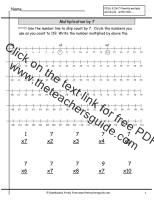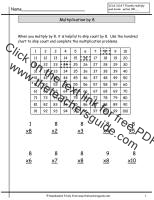Multiplication Facts WorksheetsMultiplication by zero and one Worksheet,  2

Students multiply numbers by 0 and 1.Multiplication by Two with Numberline Worksheet

Students multiply numbers by 2 with included numberline for skip counting.Multiplication by Two with Hundred Chart Worksheet

Students multiply numbers by 2 with included hundred chart for skip countingMultiplication by Two with Doubles Worksheet

Students multiply numbers by two and associate with doubles problems.Multiplication by Two Facts Worksheet

Students multiply numbers 2.Multiplication by Three With Numberline Worksheet

Students multiply numbers by 3 with included numberline for skip countingMultiplication by Three with Hundred Chart Worksheet

Students multiply numbers by 3 with included hundred chart for skip countingMultiplication by Three Worksheet

Students multiply numbers by 3.Multiplication by Four With Numberline Worksheet

Students multiply numbers by 4 with included numberline for skip countingMultiplication by Four with Hundred Chart Worksheet

Students multiply numbers by 4 with included hundred chart for skip countingMultiplication by Four Worksheet

Students multiply numbers by 4.Multiplication by Five With Numberline Worksheet

Students multiply numbers by 5 with included numberline for skip countingMultiplication by Five with Hundred Chart Worksheet

Students multiply numbers by 5 with included hundred chart for skip countingMultiplication by Five Worksheet,

Students multiply numbers by 5.Multiplication by Six With Numberline Worksheet

Students multiply numbers by 6 with included numberline for skip countingMultiplication by Six with Hundred Chart Worksheet

Students multiply numbers by 6 with included hundred chart for skip countingMultiplication by Six Worksheet

Students multiply numbers by 6.Multiplication by Seven With Numberline Worksheet

Students multiply numbers by 7 with included numberline for skip countingMultiplication by Seven with Hundred Chart Worksheet

Students multiply numbers by 7 with included hundred chart for skip countingMultiplication by Seven Worksheet

Students multiply numbers by 7.Multiplication by Eight With Numberline Worksheet

Students multiply numbers by 8 with included numberline for skip countingMultiplication by Eight with Hundred Chart Worksheet

Students multiply numbers by 8 with included hundred chart for skip countingMultiplication by Eight Worksheet

Students multiply numbers by 8.Multiplication by Nine With Numberline Worksheet

Students multiply numbers by 9 with included numberline for skip countingMultiplication by Nine with Hundred Chart Worksheet

Students multiply numbers by 9 with included hundred chart for skip countingMultiplication by Nine Worksheet

Students multiply numbers by 9.Multiplication by Ten Worksheet

Students multiply numbers by 10.Multiplication by 0-3 Worksheet0-40-50-60-70-80-90-100-10

Students multiply numbers.Multiplication by Blank Page

Choose the number students are multiplying.